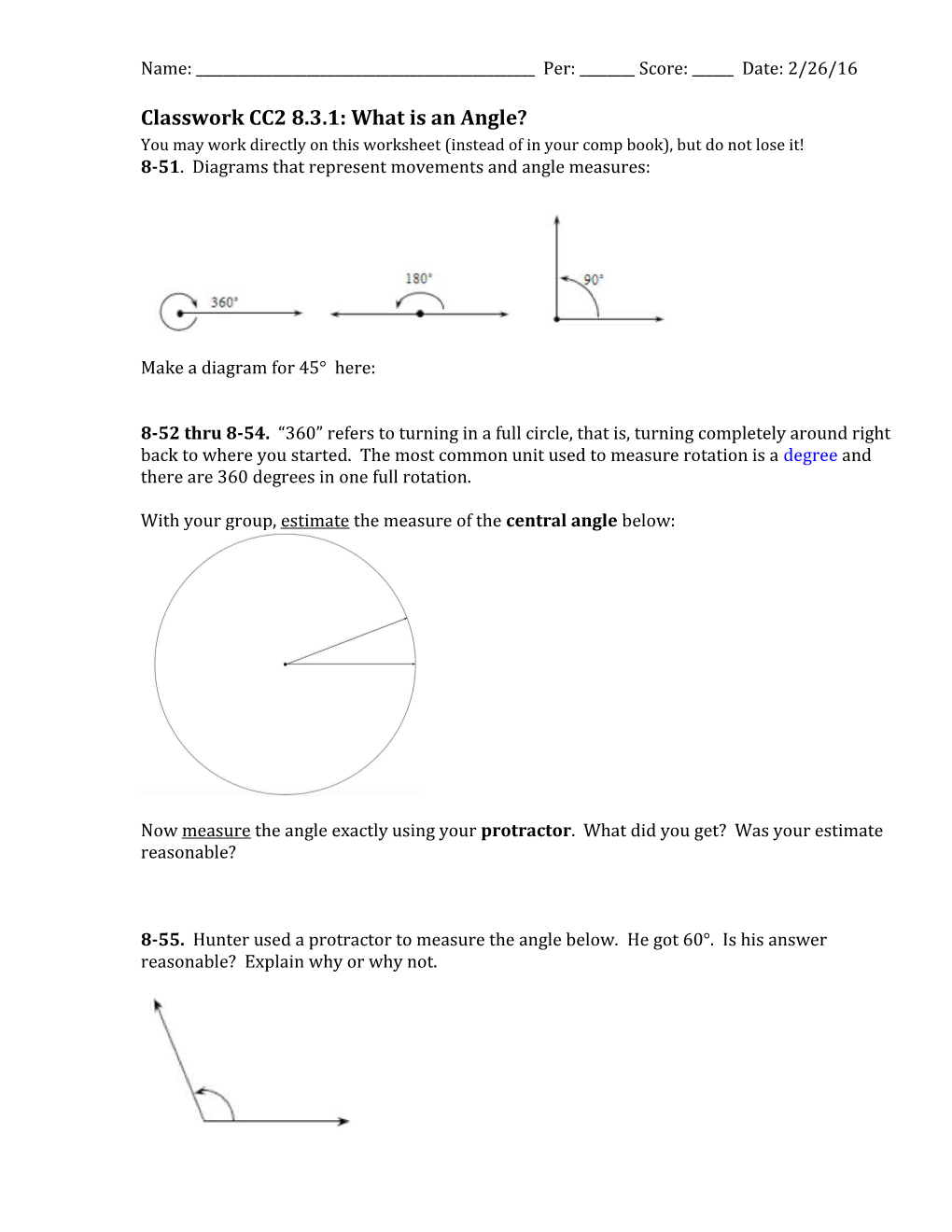# Classwork CC2 8.3.1: What Is an Angle?Name: ______Per: ______Score: ______Date: 2/26/16

Classwork CC2 8.3.1: What is an Angle?

You may work directly on this worksheet (instead of in your comp book), but do not lose it!

8-51. Diagrams that represent movements and angle measures:

Make a diagram for 45° here:

8-52 thru 8-54. “360” refers to turning in a full circle, that is, turning completely around right back to where you started. The most common unit used to measure rotation is a degree and there are 360degrees in one full rotation.

With your group, estimate the measure of the central angle below:

Now measure the angle exactly using your protractor. What did you get? Was your estimate reasonable?

8-55. Hunter used a protractor to measure the angle below. He got 60°. Is his answer reasonable? Explain why or why not.

8-56. Estimate the angles formed in the diagrams below without measuring. Be ready to explain your estimates.

8-57. Angles can be thought of as the result of a rotation or turn. They can also help you to describe shapes. First estimate, thenmeasure the indicated angles in degrees.

Notes in Toolkit.

Classwork CC2 8.3.2: Classifying Angles

If you look around you, you will see angles everywhere. Angles are important in construction, sports, and art. In the previous lesson, you learned how to measure angles using a protractor.Today you will classify angles and use your protractor to identify special angles.

8-66.In geometry, an angle is formed by two rays with a common endpoint. A ray is a line that has an endpoint and extends infinitely in a particular direction. The point where the two rays intersect is called the vertex of the angle.

One of the most common angles is a right angle. A right angle measures 90º. If an angle measures less than 90º, it is called an acute angle. If an angle measures more than 90º but less than 180º, it is called an obtuse angle.Use a protractor to find the measure of each angle below, and then classify it according to its measure.

A straight line is also called a straight angle. Draw a straight angle on your paper. What is the measure of a straight angle?

8-67. The diagrams below show some students’ use of protractors. Explain what each student needs to do to find the correct angle measure.

8-68.Complementary anglesare two angles with a sum of 90º.

Supplementary anglesare two angles with a sum of 180º.

1. Use your protractor to measure each of the angles below and determine which angles are complementary and which angles aresupplementary.
1. With your team, come up with a strategy for remembering the definitions of complementary andsupplementary.

Anglesthat share that same vertex and one side are referred to as adjacent angles. “Adjacent” in the English language means “next to.” For two angles to be adjacent, they must satisfy the following threeconditions:

1. The two angles must have a common (shared or same) side.
2. They must have a common vertex (a common starting point for all sides).
3. They can have no interior points in common (no overlap is permitted). The common side must be between the two angles.

Look at the angles below. Determine which pairs of angles are adjacent and which are not by checking to see if each angle pair satisfies all three conditions. Write “yes” or “no” next to each figure. If you write “no,” give a short explanation.

8-70. VERTICAL ANGLES

Angles formed by two intersecting lines that are not adjacent are called vertical angles. In the diagram below, angles 1 and 2 are vertical angles.

1. What can you say about angles 3 and 4?
2. Use your protractor to measure each of the four angles. What do you notice?
3. Draw a pair of vertical angles on your paper. Each team member should draw angles with different measures. Measure your vertical angles. What do you think is true about the measures of vertical angles?

Notes in Toolkit.

8-71. Find the measure of the missing angles. Show your work.

a. / / b. /
c. / / d. /

8-72.The problems below describe other situations where some angles have been put together to create larger angles. Foreach problem below, write an algebraic equation to represent the situation and then solve algebraically to answer the question.

a) Three angles that all have the same measure and one 36º angle combine to make a 90°angle, as shown in thediagram at right. Whatis the measure of each of thethree unmarked angles?

b) Additional Challenge:One angle and two other angles that are each half aslarge as the first combine to create an angle that measures 140°, as shown in the diagram at right. What are the measures of each of the angles?

8-73. Additional Challenge: Determine the size of all of the angles labeled with variables. Show your work where possible.